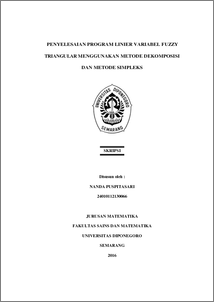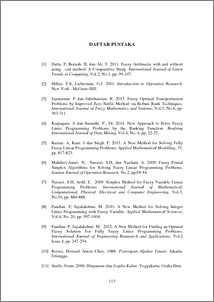# PENYELESAIAN PROGRAM LINIER VARIABEL FUZZY TRIANGULAR MENGGUNAKAN METODE DEKOMPOSISI DAN METODE SIMPLEKS

PUSPITASARI, NANDA (2016) PENYELESAIAN PROGRAM LINIER VARIABEL FUZZY TRIANGULAR MENGGUNAKAN METODE DEKOMPOSISI DAN METODE SIMPLEKS. Undergraduate thesis, UNDIP.Preview
PDF
179KbPDF
Restricted to Repository staff only

14MbPreview
PDF
16Kb

## Abstract

Fuzzy Variable Linear Programming (FVLP) with triangular fuzzy variable is part of not fully fuzzy linear programming with decision variables and the right side is a fuzzy number. Solving FVLP with triangular fuzzy variables used Decomposition Methods and Simplex Methods or Big-M Methods by using Robust Ranking to obtain crisp values. Decomposition Methods of resolving cases maximization and minimization FVLP by dividing the problems into three parts CLP. Solving FVLP with Simplex method for maximizing case and Big-M Methods to directly solve the minimization case FVLP do without confirmation first. The optimal solution fuzzy, crisp optimal solution, optimal objective function fuzzy and crisp optimal objective function generated from Decomposition Methods and Simplex Methods for maximizing case has same solution. So as Decomposition Methods and Big-M Methods for minimizing case has same solution. Decomposition Methods has a longer process because it divides the problem into three parts CLP and Simplex Methods or Big-M Methods has a fewer processes but more complicated because the process without divide the problems into three parts. Keywords : Not Fully Fuzzy Linear Programming, Triangular Fuzzy Variables, Triangular Fuzzy Number, Decomposition Methods, Simplex Methods, Big-M Methods, Robust Ranking.

Item Type: Thesis (Undergraduate) Q Science > QA Mathematics Faculty of Science and Mathematics > Department of Mathematics 84279 INVALID USER 14 Jun 2022 09:01 14 Jun 2022 09:01

Repository Staff Only: item control page